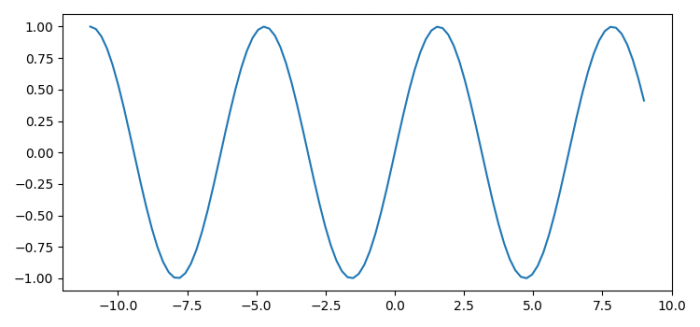# Scroll backwards and forwards through Matplotlib plots

To scroll backward and forwards (left and right keys) through Matplotlib plots, we can take the following steps −

• Set the figure size and adjust the padding between and around the subplots.
• Create curr_pos and y using numpy.
• Create a new figure or activate an existing figure using figure() method.
• Bind the function to the event, i.e., key_press_event.
• Add an '~.axes.Axes' to the figure as part of a subplot arrangement.
• Plot curr_pos and y data points using plot() method.
• If the left and right arrow keys could be used, then the curve could go right and left accordingly.
• To display the figure, use show() method.

## Example

import numpy as np
import matplotlib.pyplot as plt

plt.rcParams["figure.figsize"] = [7.50, 3.50]
plt.rcParams["figure.autolayout"] = True

curr_pos = np.linspace(-10, 10, 100)
y = np.sin(curr_pos)

def key_event(e):
global curr_pos
if e.key == "right":
curr_pos = curr_pos + 1
else:
curr_pos = curr_pos - 1
ax.cla()
ax.plot(curr_pos, np.sin(curr_pos))
fig.canvas.draw()
fig = plt.figure()
fig.canvas.mpl_connect('key_press_event', key_event)

plt.show()